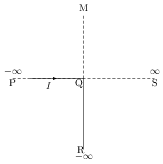# Magnetic Field Near a Current Carrying Straight Wire

## Problems from IIT JEE

Problem (IIT JEE 2000):An infinitely long conductor PQR is bent to form a right angle as shown in figure. A current $I$ flows through PQR. The magnetic field due to this current at the point M is $B_1$. Now, another infinitely long straight conductor QS is connected at Q, so that current is $I/2$ in QR as well as in QS, the current in PQ remaining unchanged. The magnetic field at M is now $B_2$. The ratio $B_1/B_2$ is given by

1. 1/2
2. 1
3. 2/3
4. 2

Solution:The magnetic field at the point P (see figure) by a current carrying wire is given by \begin{alignat}{2} &B=\frac{\mu_0I}{4\pi d}(\cos\theta_1-\cos\theta_2).\nonumber \end{alignat} In first case, the field at the point M by part PQ, part QR, and total field are given by \begin{alignat}{2} &B_\text{PQ}=\frac{\mu_0I}{4\pi d}(\cos 0-\cos 90)=\frac{\mu_0I}{4\pi d}, \nonumber\\ &B_\text{QR}=\frac{\mu_0I}{4\pi d}(\cos 180-\cos 180)=0,\nonumber\\ &B_1=B_\text{PQ}+B_\text{QR}=\frac{\mu_0I}{4\pi d}. \nonumber \end{alignat} In second case, the field at M by part PQ and part QR remains same as in first case. The field by part QS and total field are given by, \begin{alignat}{2} &B_\text{QS}=\frac{\mu_0(I/2)}{4\pi d}(\cos90-\cos 180)=\frac{\mu_0I}{8\pi d}, \nonumber \\ &B_2=B_\text{PQ}+B_\text{QR}+B_\text{QS}=\frac{3}{2}\frac{\mu_0I}{4\pi d}. \nonumber \end{alignat}